Monday 08th August 2022CBSE Guess > Papers > Important Questions > Class XI > 2010 > Maths > Maths By Mr. Anil Kumar Tondak CBSE CLASS XI
Principle of Mathematical InductionProve the followings by using Principle of Mathematical Induction: Q.1. 1 +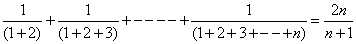. Q.2.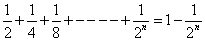Q.3.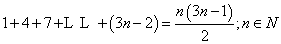Q.4. 1 + 2 + 3 +-------+ n =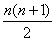Q.5 . . 12 + 32 + 52 +-----------+(2n – 1)2 =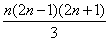Q.6. 1.3 + 2.4 + 3.5 +--------+n.(n+2) = 1/6 n(n+1)(2n+7) Q.7.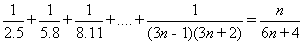. Q.8. 1.2.3 + 2.3.4 + … + n (n + 1) (n+2) =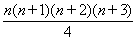for all n ÎN. Q.9.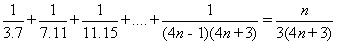Q.10.Q.11.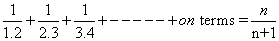Q.12. 1 + 4 + 7 +-------+(3n-2) =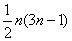Q.13. 12 + 22 + 32 +---------+ to n term =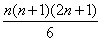. Q.14. 3.6 + 6.9 + 9.12 +--------+3n(3n+3) =3n (n+1) (n+2) Q.15.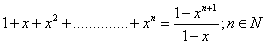Q.16.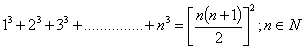Q.17. 1.3 + 2.32 + 3.32 + … + n.3n =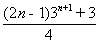for all n ÎN. Q.18. a + ar + ar2+----------+arn-1=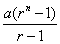Q.19. Prove : 102n-1 + 1 is divisible by 11. Q.20. Prove : 2.7n + 3.5n – 5 is divisible by 24 for all n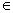N Q.21. Prove: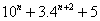is divisible by 9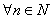Q.22. Prove: 52n – 1 is divisible by 24 for all n ÎN. Q.23. Prove: 32n + 7 is divisible by 8 for all n ÎN. Q.24. Prove: 52n+2 – 24n – 25 is divisible by 576 for all n ÎN. Q.25. Prove: 72n + 23n – 3 , 3n-1 is divisible by 25 for all n ÎN. Q.26. Prove: n3 + (n + 1)3 + (n – 2)3 is a multiple of 9 Q.27. Prove: 4n + 15n – 1 is divisible by 9. Q.28. Prove: 23n – 1 is divisible by 7 Q.29. Prove: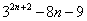is divisible by 64 for every natural number n . Q.30. Prove: 2.7n + 3.5n – 5 is divisible by 24 for all n ÎN. Q.31. Prove: 11n + 2 + 122n + 1 is divisible by 133 for all n ÎN. Q.32. Prove: x2n-1 + y2n-1 is divisible by x + y for all n ÎN. Q.33. Prove : x2n-1 is divisible by (x – 1) Q.34. Prove by mathematical induction that 41n – 14n is a multiple of 27. Q.35. Prove : 1 + 2 + 3 … + n <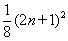for all n ÎN. Q.36 .Prove: 12 + 22 +----------+n2 >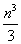, nN. Q.37. Prove: 3n > n for all nN Q.38. Prove the rule of exponents (ab)n = an bn Q.39. Prove by the principle of mathematical induction that: n (n+1) (2n+1) is divisible by 6 for all n Î N Q.40. If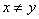then prove that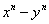is divisible by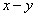for every natural number n. Q.41 . Prove by induction that (2n + 7) < (n + 3)2 for all natural numbers n. Using this, prove by induction that (n + 3)2 £ 2n+3 for all n ÎN. Paper By Mr. Anil Kumar Tondak Email Id : [email protected] Ph No.: 9811363962